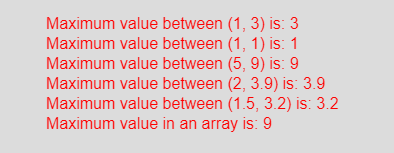# p5.js | max() function

The max() function in p5.js is used to get the maximum value among sequence of numbers or two given numbers. The max() function accepts any number of parameters or an array.

Syntax:

`max(a, b)`

or

`max(arr)`

Parameters: The max(a, b) function accepts two parameters which are different number and compared to get maximum value among them. The max(arr) function accepts an array of numbers and returns the maximum value.

Return Value: It returns the maximum value between the numbers.

Below program illustrates the max() function in p5.js:

Example: This example uses max() function to get the maximum value.

 `function` `setup() {  ` `  `  `    ``// Create Canvas of given size ` `    ``createCanvas(450, 230);  ` `}  ` `  `  `function` `draw() {  ` `      `  `    ``// Set the background color  ` `    ``background(220);  ` `      `  `    ``// Call to max() function  ` `    ``let u = max(1, 3); ` `    ``let v = max(1, 1); ` `    ``let w = max(5, 9); ` `    ``let x = max(2, 3.9); ` `    ``let y = max(1.5, 3.2); ` `     `  `    ``let arr = [1, 5, 3, 8, 6, 9]; ` `    ``let z = max(arr); ` `       `  `    ``// Set the size of text  ` `    ``textSize(16);  ` `      `  `    ``// Set the text color  ` `    ``fill(color(``'red'``));  ` `    `  `    ``// Getting minimum value     ` `    ``text(``"Maximum value between (1, 3) is: "` `+ u, 50, 30); ` `    ``text(``"Maximum value between (1, 1) is: "` `+ v, 50, 50); ` `    ``text(``"Maximum value between (5, 9) is: "` `+ w, 50, 70); ` `    ``text(``"Maximum value between (2, 3.9) is: "` `+ x, 50, 90); ` `    ``text(``"Maximum value between (1.5, 3.2) is: "` `+ y, 50, 110); ` `    ``text(``"Maximum value in an array is: "` `+ z, 50, 130); ` `           `  `}  `

Output:Reference: https://p5js.org/reference/#/p5/max

My Personal Notes arrow_drop_upCheck out this Author's contributed articles.

If you like GeeksforGeeks and would like to contribute, you can also write an article using contribute.geeksforgeeks.org or mail your article to contribute@geeksforgeeks.org. See your article appearing on the GeeksforGeeks main page and help other Geeks.

Please Improve this article if you find anything incorrect by clicking on the "Improve Article" button below.

Article Tags :

Be the First to upvote.

Please write to us at contribute@geeksforgeeks.org to report any issue with the above content.